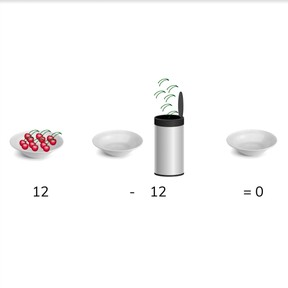Subtraction to 20 with result of 0 or 1

# Subtraction to 20 with result of 0 or 1

No account needed.8,000 schools use Gynzy92,000 teachers use Gynzy1,600,000 students use Gynzy

## General

Students learn to subtract numbers to 20 whose difference is 0 or 1.

1.OA.C

## Relevance

It is important o be able to subtract numbers to 0 or 1 to be able to quickly determine if you have 0 or 1 left.

## Introduction

Students count forward and backward to 20. Do so in a variety of manners, whispering, in a silly voice, loudly, starting quiet and getting loud, starting loud and getting quiet.

## Development

Explain to students that when you subtract a number equal to the quantity you have, you have 0 left. Show this with the example of the chickens and the chicken coop. Explain that this is subtracting to 0. Then discuss the image of candies with the students. Ask how many candies need to be taken away to subtract to 0. Next show a subtraction to 0 problem without visual support. Explain that when the number after the minus sign is the same as the number before the minus sign, that the answer is always zero.
Next explain to students that when the number you take away is 1 less than the number you have, you have 1 left. Show this with the example of the chickens and the chicken coop. Explain that this is subtraction to 1. Show the example of candies and ask how many they need to take away to subtract to 1. Next show a subtraction problem to 1 without visual support. Explain that when the number after the minus sign is 1 less than the number before the minus sign, that the answer is always 1.
Students must next determine which subtraction problems are subtraction to 0, and which are subtraction to 1. Drag the subtraction problems to the correct column. Finally do a selection of subtraction problems with the class, and make sure students understand how to determine if the subtraction problem is to 0 or 1.

To check that students understand subtraction with numbers to 10 with a result of 0 or 1 you can ask the following questions:
- What is subtraction to 0?
- What is subtraction to 1?
- Is 16-15 subtraction to 0 or 1? How do you know?

## Guided practice

Students start by practicing with subtraction to 0 and are then given problems with subtraction to 1. In the third exercise they get two subtraction problems, one to 0 and one to 1.

## Closing

Repeat the goal of the lesson and why it is important to learn how to subtract to 0 or 1. Next, play a game with the students where they must determine if a subtraction problem is to 0 or 1. If the subtraction problem is to 0, they lower their arms, if the subtraction problem is to 1, they raise their arms in the air. You can ask students to explain how they know if it is subtraction to 0 or 1.

## Teaching tips

If students have difficulty with this learning goal, they can be supported by making use of blocks or other manipulatives. They can then visually see if there are 0 left or 1 left when they take the second number away from the first.

## Instruction materials

optional: blocks or other manipulatives

### The online teaching platform for interactive whiteboards and displays in schools

• Save time building lessons

• Manage the classroom more efficiently

• Increase student engagement